# Difference between revisions of "Continuity theorem"

continuity principle

Letbe a domain of holomorphy in,, and let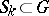and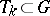,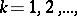be two sequences of sets, with compact closures in, for which the maximum modulus principle holds for functionsthat are holomorphic in, that is,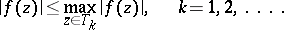Then if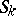converges to some bounded setand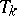to a set, and if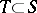andhas compact closure in, thenhas compact closure in. If forone takes analytic hypersurfaces and fortheir boundaries, one obtains the Behnke–Sommer theorem (see ). Hence it follows that every domain of holomorphy is pseudo-convex. Applied to a specific function, certain modifications of the continuity theorem are known as theorems on "analytic discs" . For example, the strong theorem on analytic "discs" asserts the following. Suppose that in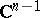a Jordan curve of the form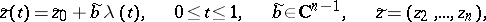is given. Let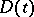,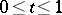, be a family of domains in the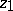-plane having the property that for any compact setthere is a numbersuch thatfor all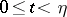. Ifis holomorphic at the points of the "discs"and at one point of the limiting "disc"thenis holomorphic also at all points of the limiting "disc" . Theorems on "analytic discs" are very useful in the holomorphic extension of domains and in constructing envelopes of holomorphy (cf. Holomorphic envelope), for example, in the proof of Bochner's theorem on the envelope of holomorphy of a tube domain, of the Osgood–Brown theorem, and of the theorem on "imbedded edges" , "the edge-of-the-wedge" , "C-convex hulls" , and others. The continuity principles given go back to the Hartogs theorem on removable singularities (1916) for holomorphic functions of several complex variables.

How to Cite This Entry:
Continuity theorem. Encyclopedia of Mathematics. URL: http://encyclopediaofmath.org/index.php?title=Continuity_theorem&oldid=24404
This article was adapted from an original article by V.S. Vladimirov (originator), which appeared in Encyclopedia of Mathematics - ISBN 1402006098. See original article